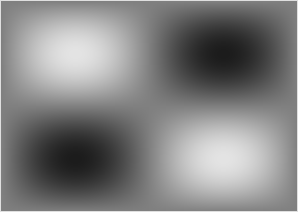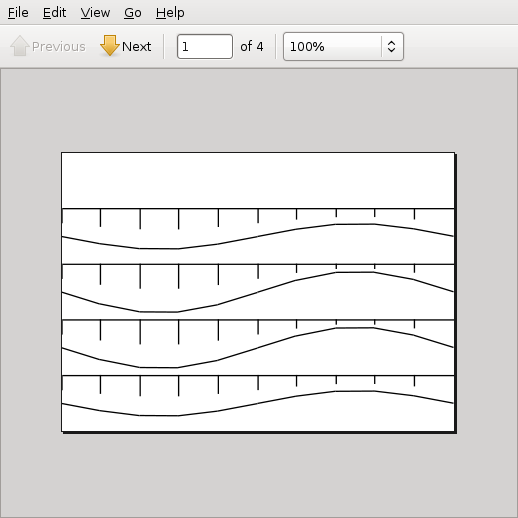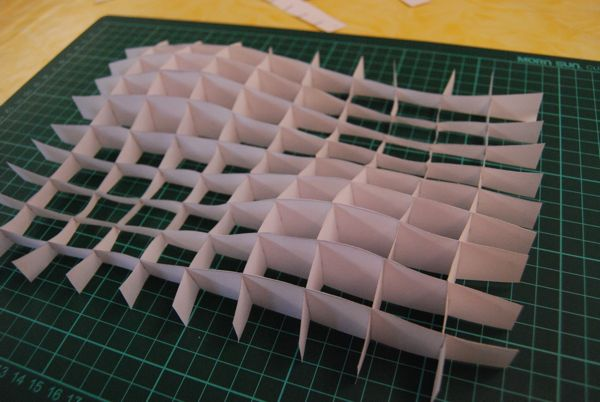## How To Make a 3D-Paper Model from a Heightfield in Processing

I just wrote a little processing sketch that takes a height field like thiscalculates a pdf containing something like thiswhich can be cut out and sticked together to a 3d model like thisHere is the code i used to render the heightfield and generate the pdf. The pdf will be stored in the sketchfolder in and named output.pdf

``````/**
A paper model generator written by <a href='https://www.local-guru.net/blog/'>Guru</a>
*/
import processing.pdf.*;

void setup() {
size(296,210);
noLoop();
}

void draw() {
// Generate heightfield
for( int x = 0; x< width; x++) {
for( int y = 0; y < width; y++) {
stroke( 128 + 100 * sin( map(x,0,width, 0, TWO_PI)) * sin(map(y,0,height,0,TWO_PI)));
point(x,y);
}
}

// Generate pdf
PGraphicsPDF pdf = (PGraphicsPDF) createGraphics(296, 210, PDF, "output.pdf");
pdf.beginDraw();
pdf.background(255);

for ( int i =1; i < 5; i++ ) {
int y = height / 10 * i;
pdf.line( 1, height/5 * i, width, height/5 * i );
for( int j = 1; j < 11; j++) {
int x1 = (j - 1 ) * width/10;
int x2 = j * width/10 -1 ;
float v1 = brightness(get(x1, y));
float v2 = brightness(get(x2, y));

pdf.line( x1, height/5 * i + map(v1,0,255,0,height/5), x2, height/5 * i +  map(v2,0,255,0,height/5) );

pdf.line( x1, height/5 * i , x1, height/5 * i + map(v1,0,255,0,height/5)/2 );
}
}

pdf.nextPage();
for ( int i =0; i < 5; i++ ) {
int y = height / 10 * (i+5);
pdf.line( 0, height/5 * i, width, height/5 * i );
for( int j = 1; j < 11; j++) {
int x1 = (j - 1 ) * width/10;
int x2 = j * width/10 -1 ;
float v1 = brightness(get(x1, y));
float v2 = brightness(get(x2, y));

pdf.line( x1, height/5 * i + map(v1,0,255,0,height/5), x2, height/5 * i +  map(v2,0,255,0,height/5) );

pdf.line( x1, height/5 * i , x1, height/5 * i + map(v1,0,255,0,height/5)/2 );
}
}

pdf.nextPage();
for ( int i =1; i < 5; i++ ) {
int x = width/ 10 * i;
pdf.line( width/5 * i, 0, width/5 * i, height );

for( int j = 1; j < 11; j++) {
int y1 = (j - 1 ) * height/10;
int y2 = j * height/10 -1 ;
float v1 = brightness(get(x, y1));
float v2 = brightness(get(x, y2));

pdf.line( width/5 * i + map(v1,0,255,0,height/5),y1, width/5 * i +  map(v2,0,255,0,height/5), y2 );

pdf.line( width/5 * i + map(v1,0,255,0,height/5),y1, width/5 * i + map(v1,0,255,0,height/5)/2,y1 );
}
}

pdf.nextPage();
for ( int i =0; i < 5; i++ ) {
int x = width/ 10 * (i+5);
pdf.line( width/5 * i, 0, width/5 * i, height );

for( int j = 1; j < 11; j++) {
int y1 = (j - 1 ) * height/10;
int y2 = j * height/10 -1 ;
float v1 = brightness(get(x, y1));
float v2 = brightness(get(x, y2));

pdf.line( width/5 * i + map(v1,0,255,0,height/5),y1, width/5 * i +  map(v2,0,255,0,height/5), y2 );

pdf.line( width/5 * i + map(v1,0,255,0,height/5),y1, width/5 * i + map(v1,0,255,0,height/5)/2,y1 );
}
}

pdf.dispose();
pdf.endDraw();
}
``````